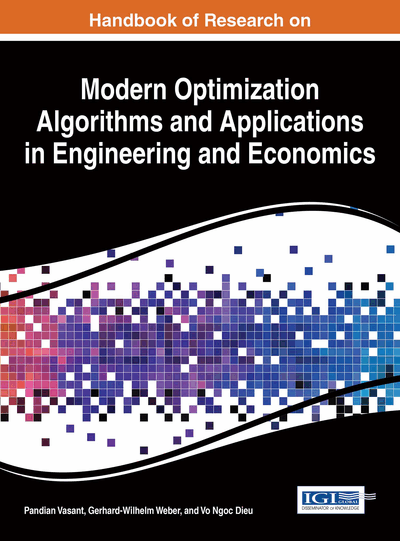# Robust Two-Stage and Multistage Optimization: Complexity Issues and Applications

Michel Andre Minoux (University P. and M. Curie, France)
DOI: 10.4018/978-1-4666-9644-0.ch002
Available
\$37.50
No Current Special Offers

## Abstract

This chapter is intended as an overview of robust optimization models related to optimization problems subject to uncertain data, with special focus on the case when uncertainty impacts the right-hand side coefficients in the constraints. Two-stage as well as multistage models are addressed, emphasizing links with applications and computational complexity issues. A class of multistage robust optimization problems for which exact optimal strategies can be efficiently computed (via a robust dynamic programming recursion) is discussed. An application to a multiperiod energy production planning problem is presented into detail, and computational results are reported.
Chapter Preview
Top

## 2. Background: Robust 2-Stage (Linear) Optimization Problems

In this section we fist introduce a generic model for the class of robust 2-stage (linear) optimization problems featuring right-hand side uncertainty (RHS-uncertainty). We next discuss links with related stochastic models and illustrate how the two approaches differ in their capability of taking risk concerns into account. Finally, we provide an overview of possible applications, emphasizing known complexity (NP-hardness) results and polynomial-time solvable special cases.

## Key Terms in this Chapter

Linear Programming Problem: A linear programming (LP) problem is an optimization problem which consists in finding the maximum or the minimum value of a given linear function of n real decision variables subject to a given set of linear equality or inequality constraints.

Robust Optimal Solution: We define this here for a two-stage minimization problem with decision variables x, recourse variables y, and depending on u, a p-component vector the components of which represent the various parameters subject to uncertainty; assuming that u is known to belong to some given (finite or compact) uncertainty set U in p-dimensional space, the value h(x,v) of any solution x in case u=v (with v an element of U) is the minimum objective function value achievable by adjusting the recourse variables y. The worst-case value w(x) of any given solution x is then defined as the maximum taken over v in U of h(x,v), and an optimal robust solution x* is a solution minimizing w(x). It is thus realized that such a x* is obtained as the result of a Min-Max optimization process. The problem of minimizing w(x) is called the deterministic equivalent to the robust optimization problem under consideration.

Multistage Robust or Stochastic Optimization: This refers to an optimization problem with uncertain or random parameters, the realizations of which are revealed progressively over successive time periods (or stages). For a k-stage instance, the set of decision variables is decomposed into (x 1 , y 1 , x 2 , y 2 , . .., x k , y k ); in any stage t, the decision variables x t have to be fixed knowing the realizations of the uncertain or random parameters in stages 1, 2, … t-1, but prior to getting any information about the realization of the uncertain or random parameters in stage t and in subsequent stages; the decision variables y t can be adjusted after observing the realized values of the uncertain or random parameters in stages 1, 2, .., t.

Right-Hand Side Uncertainty: An optimization problem featuring right-hand side uncertainty corresponds to the situation when the only uncertain parameters correspond to all (or part of) the right-hand side coefficients in the constraints.

Two-Stage Robust or Stochastic Optimization: This refers to an optimization problem with uncertain or random parameters, in which the set of decision variables (x, y) is decomposed into two distinct subsets: the set of primary (or 'here and now') decision variables x, and the set of secondary (or 'wait-and-see') variables y; the former have to be fixed prior to getting any information about the realization of the uncertain or random parameters, while the latter can be adjusted after observing the realized values of the uncertain or random parameters.

Polyhedral Uncertainty Set: This corresponds to the special case (frequently arising in applications) of an uncertainty set defined as the set of solutions to a given linear equality/inequality system.

Uncertainty Set: This is one of the basic input data which is required to specify an optimization problem under uncertainty on some of the parameters such as coefficients in the constraints or in the objective function. Given p uncertain real parameters, the uncertainty set (in p-dimensional real space) is the set of values which can possibly be taken by the p parameters considered. This set may feature finite cardinality, or may contain infinitely many elements (compactness being assumed in this case) .

Stochastic Optimization Problem: We define this here for a two-stage minimization problem with decision variables x, recourse variables y, and depending on u, a p-component vector the components of which represent the various random parameters assumed to follow a given probability distribution in p-dimensional space; the value h(x,v) of any solution x in case u=v arises, is the minimum objective function value achievable by adjusting the recourse variables y. The expected value of any given solution x is then defined as the expectation of h(x,v) with respect to the probability distribution of v, and an optimal solution to the stochastic optimization problem is a solution x* minimizing this expected value.

## Complete Chapter List

Search this Book:
Reset